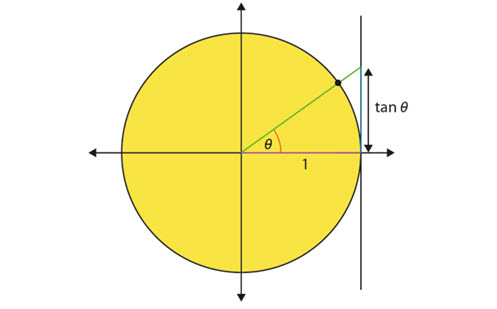# 3.2 Trigonometry

This paper introduces trigonometry by breaking it into two sections:

• Understanding trigonometric functions
• Using trigonometry to solve problems in context.

It provides details of the knowledge students should have and some of the misconceptions they may hold. Examples are given to illustrate what these misconceptions may look like, how to address them, and support teachers in developing pedagogical and content knowledge.

Year level(s) Year 9, Year 10
Purpose Content knowledge
Keywords Trigonometry, ratio, sine, cosine, tangent

## Curriculum alignment

Strand and focus Space
Topics Pythagoras and trigonometry
AC: Mathematics (V9.0) content descriptions
AC9M9SP02
Apply the enlargement transformation to shapes and objects using dynamic geometry software as appropriate; identify and explain aspects that remain the same and those that change

AC9M9M03

Solve spatial problems, applying angle properties, scale, similarity, Pythagoras' theorem and trigonometry in right-angled triangles

AC9M10M03

Solve practical problems applying Pythagoras' theorem and trigonometry of right-angled triangles, including problems involving direction and angles of elevation and depression

Numeracy progression Understanding geometric properties (P7)
Proportional thinking (P7)
Understanding units of measurement (P10)